• 余弦相似度计算matlab代码计算余弦相似度 张伯超 该脚本将从免疫数据库中读取数据并计算不同特征样本之间的余弦相似度。 用法 -d name of database -s name of subject -f field of the columns used to separate ...
• 衡量两个向量x、y的相关性(夹角的余弦) MATLAB 代码余弦相似度也被⼀些迁移学习研究所使用。
• 文本聚类 文本聚类的一种实现，使用 k-means 进行聚类，并使用作为距离度量。 等等，什么？ 基本上，如果您有一堆文本文档，并且您想按相似性将它们分成 n 个组，那么您很幸运。 例子 为了测试这一点，我们可以查看...Python
• Matlab余弦相似度算法判断图片相似度并识别源代码 Matlab 余弦相似度 图像匹配 可直接运行
• 文档所给的是余弦相似度计算代码，在文本分类中用来计算相似度值，以此对文本分类
• matlab中余弦相似度代码用于盲语音分离的定向稀疏滤波。 以下论文的 MATLAB 代码： K. Watcharasupat, AHT Nguyen, C. -H. Ooi 和 AWH Khong，“使用加权 Lehmer 均值进行不平衡语音混合盲分离的定向稀疏滤波”，...
• matlab中余弦相似度代码vgg_face_matconvnet VGG 人脸描述符源代码和模型 (MatConvNet) 这是我学习 NN & DL 的起点。我想与伟大的 Github 社区分享学习经验。 我利用预训练模型来完成人脸验证任务。结果是两个人脸的...
• 用2.7版本Python实现比较2个文档的相似度计算，采用余弦相似度方法，main函数中的fileName1和fileName2是自己构造的数据集，把这几个文档放在一起就可以运行。
• n_row = size(A,1); norm_r = sqrt(sum(abs(A).^2,2)); % same as norm(A,2,'rows') B = zeros(n_row,n_row); for i = 1:n_row for j = i:n_row ...如果大家有了解改进的余弦相似度的，欢迎交流哈~ ...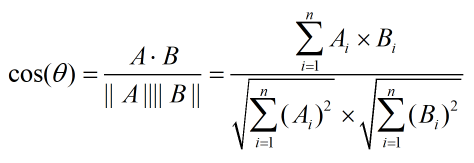n_row = size(A,1);
norm_r = sqrt(sum(abs(A).^2,2)); % same as norm(A,2,'rows')
B = zeros(n_row,n_row);
for i = 1:n_row
for j = i:n_row
B(i,j) = dot(A(i,:), A(j,:)) / (norm_r(i) * norm_r(j));
B(j,i) = B(i,j);
end
end
如果大家有了解改进的余弦相似度的，欢迎交流哈~
展开全文余弦相似度和adjust 余弦相似度 度量两个信息实体之间的相似性或距离是所有信息发现任务(无论是IR还是数据挖掘)的核心需求。采用适当的措施不仅可以提高信息选择的质量，而且有助于减少时间和处理成本。这些措施可能会被使用，例如，剽窃检测。余弦相似度是文本挖掘和信息检索中常用的基于向量的相似度度量方法。该方法将比较字符串转化为向量空间，利用欧几里德余弦规则计算相似度。这种方法通常与其他方法相结合来限制向量空间的维数。余弦相似度是内积空间中两个非零向量之间的相似度的度量，度量它们之间夹角的余弦值。例如，在信息检索和文本挖掘中，每个术语在理论上被分配一个不同的维度，而一个文档的特征是一个向量，其中每个维度的值对应于该术语在文档中出现的次数。然后，余弦相似性给出了一个有用的度量方法，来衡量两个文档在主题方面有多相似。皮尔逊相似度度量是基于一对物品的普通用户的评分与这些物品的平均评分的偏离程度。调整余弦相似度度量是基于向量的相似度的一种改进形式，我们考虑到不同的用户有不同的评分方案;换句话说，一些用户可能会对条目进行高评分，而另一些用户可能会将较低的评分作为首选。为了从基于向量的相似性中消除这个缺陷，我们从每个用户对相关项的评分中减去每个用户的平均评分。余弦方法为度量提供了一种类型的贝叶斯正则化，确保相似性不完全由两个用户共有的项的子集(可能很小)决定。这在皮尔逊相关性中并不存在(尽管它可以通过显著性加权来添加)，因此，在用户个人资料中往往有非常不同的条目集的上下文中，皮尔逊(未经纠正的)的表现原则上会更差。皮尔逊相关就是减去平均值后的余弦相似度。这很重要，因为均值代表总体积。如果总体积是有实质意义的，那么您将希望使用余弦相似性(或某种保持总体积的度量)。然而，通常它并没有什么实质性的意义，删除它是可取的。“调整余弦”相似性是通过在计算余弦公式之前减去平均值来实现的。从这个意义上说，调整后的余弦函数与皮尔逊相关函数有相同的数学公式。然而，如果公式应用不同，即使用的评级集不相同，则结果将不匹配。
展开全文• Python编写的双2D,2DPCA,...余弦相似度，https://blog.csdn.net/u012505617/article/details/89191158 feret人脸库，200人，每人7张；看到大家需要，我就上传了。代码都是我整理的，编写通过的。Oracle数据库之前上传过
• 这个值称为余弦相似度，该值在 0,1 之间变化。 因此，接近 0 (cos 90) 的向量与接近 1 (cos 0) 的向量不太相似。 执行 我们使用 scikit 的 TfidfVectorizer 将推文投影为向量，并计算这些向量之间的余弦相似度并...Python
• 余弦相似度算法: 余弦相似性通过测量两个向量的夹角的余弦值来度量它们之间的相似性。0度角的余弦值是1，而其他任何角度的余弦值都不大于1；并且其最小值是-1。从而两个向量之间的角度的余弦值确定两个向量是否...
余弦相似度算法:
余弦相似性通过测量两个向量的夹角的余弦值来度量它们之间的相似性。0度角的余弦值是1，而其他任何角度的余弦值都不大于1；并且其最小值是-1。从而两个向量之间的角度的余弦值确定两个向量是否大致指向相同的方向。两个向量有相同的指向时，余弦相似度的值为1；两个向量夹角为90°时，余弦相似度的值为0；两个向量指向完全相反的方向时，余弦相似度的值为-1。这结果是与向量的长度无关的，仅仅与向量的指向方向相关。余弦相似度通常用于正空间，因此给出的值为-1到1之间。
坐标系表示：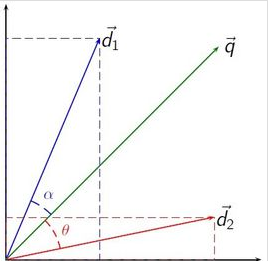具体公式: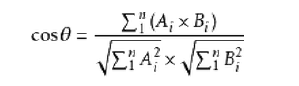具体实现(Java)：
import java.util.ArrayList;

public class SimilarityUtil {

public static double similarity(ArrayList va, ArrayList vb) {
if (va.size() > vb.size()) {
int temp = va.size() - vb.size();
for (int i = 0; i < temp; i++) {
}
} else if (va.size() < vb.size()) {
int temp = vb.size() - va.size();
for (int i = 0; i < temp; i++) {
}
}

int size = va.size();
double simVal = 0;

double num = 0;
double den = 1;
double powa_sum = 0;
double powb_sum = 0;
for (int i = 0; i < size; i++) {
double a = Double.parseDouble(va.get(i).toString());
double b = Double.parseDouble(vb.get(i).toString());

num = num + a * b;
powa_sum = powa_sum + (double) Math.pow(a, 2);
powb_sum = powb_sum + (double) Math.pow(b, 2);
}
double sqrta = (double) Math.sqrt(powa_sum);
double sqrtb = (double) Math.sqrt(powb_sum);
den = sqrta * sqrtb;

simVal = num / den;

return simVal;
}
}

案例分析:
姓名/兴趣  吃苹果  逛商店  看电视剧  打羽毛球  吃桔子  小红  3.5  5  5  5  0                    xxx  3.5  5  5  0  0
分别以小红和xxx的兴趣评分组成向量va和vb，运用多维余弦相似公式，设向量 A =
(A1,A2,...,An)，B = (B1,B2,...,Bn) ：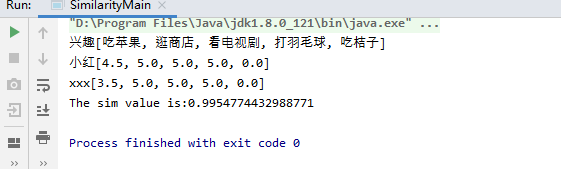计算得相似度为:0.9954774432988771
具体实现代码：
import java.util.ArrayList;

public class SimilarityMain {

public static double similarity(ArrayList va, ArrayList vb) {
if (va.size() > vb.size()) {
int temp = va.size() - vb.size();
for (int i = 0; i < temp; i++) {
}
} else if (va.size() < vb.size()) {
int temp = vb.size() - va.size();
for (int i = 0; i < temp; i++) {
}
}

int size = va.size();
double simVal = 0;

double num = 0;
double den = 1;
double powa_sum = 0;
double powb_sum = 0;
for (int i = 0; i < size; i++) {
double a = Double.parseDouble(va.get(i).toString());
double b = Double.parseDouble(vb.get(i).toString());

num = num + a * b;
powa_sum = powa_sum + (double) Math.pow(a, 2);
powb_sum = powb_sum + (double) Math.pow(b, 2);
}
double sqrta = (double) Math.sqrt(powa_sum);
double sqrtb = (double) Math.sqrt(powb_sum);
den = sqrta * sqrtb;

simVal = num / den;

return simVal;
}

public static void main(String[] args) {
String item[] = {"吃苹果", "逛商店", "看电视剧", "打羽毛球", "吃桔子"};
float a[] = {(float) 4.5, 5, 5, 5,0};
float b[] = {(float) 3.5, 5, 5, 5,0};
ArrayList vitem = new ArrayList();
ArrayList<Float> va = new ArrayList();
ArrayList<Float> vb = new ArrayList();
for (int i = 0; i < a.length; i++)
{
}
System.out.print("兴趣");
System.out.println(vitem);
System.out.print("小红");
System.out.println(va);
System.out.print("xxx");
System.out.println(vb);

SimilarityMain sim = new SimilarityMain();

double simVal = sim.similarity(va, vb);

System.out.println("The sim value is:" + simVal);
}

}

源码gitee地址:
https://gitee.com/jockhome/
展开全文• 余弦相似度算法写的c++程序，计算如“我 爱 看 电视”和“我 不 爱 看 电影”之间的余弦相似度。由于没有分词程序，所以需要用空格手动分词
• 余弦相似度基本思路是：如果这两句话的用词越相似，它们的内容就应该越相似。因此，可以从词频入手，计算它们的相似程度。 第一步，预处理主要是进行中文分词和去停用词，分词。 第二步，列出所有的词。 第三步，...

余弦相似度基本思路是：如果这两句话的用词越相似，它们的内容就应该越相似。因此，可以从词频入手，计算它们的相似程度。
第一步，预处理主要是进行中文分词和去停用词，分词。
第二步，列出所有的词。
第三步，计算词频。
第四步，写出词频向量。

余弦值越接近1，就表明夹角越接近0度，也就是两个向量越相似，这就叫"余弦相似性"。
余弦相似度缺陷
这类算法没有很好地解决文本数据中存在的自然语言问题，即同义词和多义词。这样对于搜索的精度产生很大的影响。

衡量文本相似度的几种手段：
（1）最长公共子串（基于词条空间）
（2）最长公共子序列（基于权值空间、词条空间）
（3）最少编辑距离法（基于词条空间）
（4）汉明距离（基于权值空间）
（5）余弦值（基于权值空间）

向量空间余弦相似度(Cosine Similarity)
余弦相似度用向量空间中两个向量夹角的余弦值作为衡量两个个体间差异的大小。余弦值越接近1，就表明夹角越接近0度，也就是两个向量越相似，这就叫"余弦相似性"。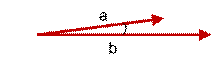上图两个向量a,b的夹角很小可以说a向量和b向量有很高的的相似性，极端情况下，a和b向量完全重合。如下图：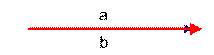如上图二：可以认为a和b向量是相等的，也即a，b向量代表的文本是完全相似的，或者说是相等的。如果a和b向量夹角较大，或者反方向。如下图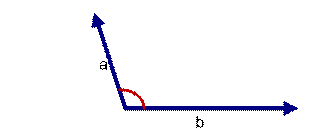如上图三: 两个向量a,b的夹角很大可以说a向量和b向量有很底的的相似性，或者说a和b向量代表的文本基本不相似。那么是否可以用两个向量的夹角大小的函数值来计算个体的相似度呢？
向量空间余弦相似度理论就是基于上述来计算个体相似度的一种方法。下面做详细的推理过程分析。
想到余弦公式，最基本计算方法就是初中的最简单的计算公式，计算夹角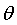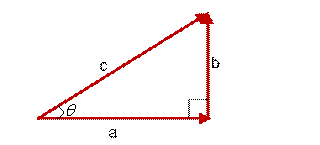图(4)
的余弦定值公式为：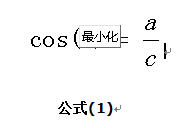但是这个是只适用于直角三角形的,而在非直角三角形中,余弦定理的公式是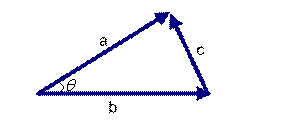图(5)
三角形中边a和b的夹角 的余弦计算公式为：公式(2)
在向量表示的三角形中，假设a向量是（x1, y1），b向量是(x2, y2)，那么可以将余弦定理改写成下面的形式：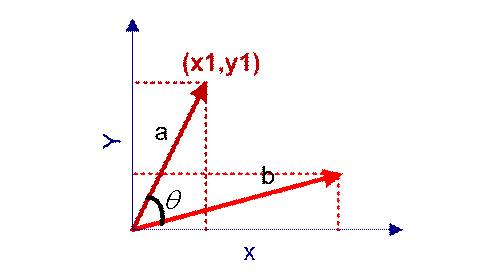图(6)
向量a和向量b的夹角 的余弦计算如下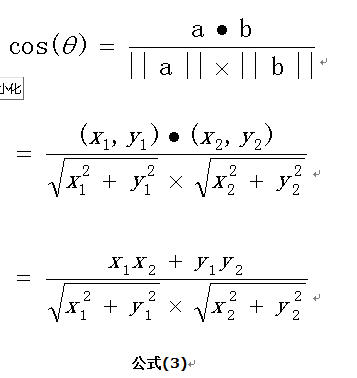扩展，如果向量a和b不是二维而是n维，上述余弦的计算法仍然正确。假定a和b是两个n维向量，a是  ，b是  ，则a与b的夹角 的余弦等于：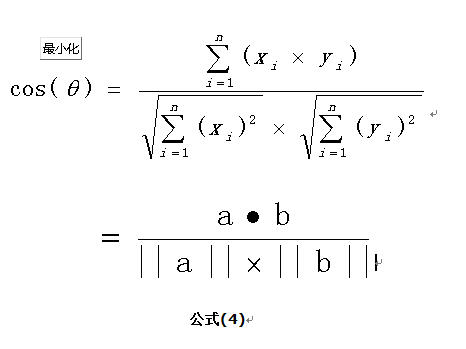余弦值越接近1，就表明夹角越接近0度，也就是两个向量越相似，夹角等于0，即两个向量相等，这就叫"余弦相似性"。
二、余弦相似度代码如下：
import java.util.HashMap;
import java.util.Map;
import java.util.Set;

/**
* 字符串相似性匹配算法
*/
public class CosDemo {
//数据结构解析:<单词,二维数组>,其中单词表示公共词，
//  二维数组一维度表示句子一的向量,另一维度表示句子二的向量
Map<Character, int[]> vectorMap = new HashMap<Character, int[]>();

int[] tempArray = null;

public CosDemo(String string1, String string2) {

for (Character character1 : string1.toCharArray()) {
if (vectorMap.containsKey(character1)) {
vectorMap.get(character1)++;
} else {
tempArray = new int;
tempArray = 1;
tempArray = 0;
vectorMap.put(character1, tempArray);
}
}
for (Character character2 : string2.toCharArray()) {
if (vectorMap.containsKey(character2)) {
vectorMap.get(character2)++;
} else {
tempArray = new int;
tempArray = 0;
tempArray = 1;
vectorMap.put(character2, tempArray);
}
}

for (Map.Entry<Character, int[]> entry : vectorMap.entrySet()) {
System.out.println("Key = " + entry.getKey() + ", Value = " + entry.getValue() +","+entry.getValue());
}
}
// 求余弦相似度
public double sim() {
double result = 0;
result = pointMulti(vectorMap) / sqrtMulti(vectorMap);
return result;
}

private double sqrtMulti(Map<Character, int[]> paramMap) {
double result = 0;
result = squares(paramMap);
result = Math.sqrt(result);
return result;
}

// 求平方和
private double squares(Map<Character, int[]> paramMap) {
double result1 = 0;
double result2 = 0;
Set<Character> keySet = paramMap.keySet();
for (Character character : keySet) {
int temp[] = paramMap.get(character);
result1 += (temp * temp);
result2 += (temp * temp);
}
return result1 * result2;
}

// 点乘法
private double pointMulti(Map<Character, int[]> paramMap) {
double result = 0;
Set<Character> keySet = paramMap.keySet();
for (Character character : keySet) {
int temp[] = paramMap.get(character);
result += (temp * temp);
}
return result;
}

public static void main(String[] args) {

String s1 = "我爱北京甜安门";
String s2 = "我喜欢吃北京烤鸭";
//第一步，预处理主要是进行中文分词和去停用词，分词。

//第二步，列出所有的词。
//公共词 ：我爱北京甜安门喜欢吃烤鸭

//第三步，计算词频，写出词频向量。
//向量1：<1,1,1,1,1,1,1,0,0,0,0,0>
//向量2：<1,0,1,1,0,0,0,1,1,1,1,1>
// 3/6 > cos =3/根号42 > 3/7即结果在3/6和3/7之间
CosDemo similarity = new CosDemo(s1, s2);
System.out.println(similarity.sim());
}

}展开全文JAVA
• 修正余弦相似度，指中心化（减去平均值）后再求余弦相似度。1. 从数学角度看，给定向量X和Y，Pearson相关系数和修正余弦相似度的计算是相同的，它们的关系参考下面的链接: ...协同过滤
•pytorch
• 该算法是用于文本相似的判定，同时也可以判定两个用户的相似性。算法是以C#实现的，封装完毕，如有急要可以留言哦
• % 计算空间向量余弦相似度 n=length(x); s1=0; for i= 1:n s1=s1+x(i)*y(i); end s2=0; for j=1:n s2=s2+x(j)*x(j); end s2=s2^0.5; s3=0; for k=1:n s3=s3+y(k)y(k); end s3=s3^0.5; s=s1/(s2s3); end ...
• 余弦相似度 from sklearn.metrics.pairwise import cosine_similarity a = [[1, 3, 2,5,6,7], [2, 2, 1,3,4,3],[2,2,2,2,2,2]] b = cosine_similarity(a) print(b) from sklearn.metrics.pairwise import cosine_...
• 距离准则 在聊接下去的内容之前，我们首先要了解一个概念，叫距离准则： 距离准则有欧氏距离，Jaccard相似度，余弦相似度，Pearson相似度这几种，我们来分别解释下。 欧式距离就是指在m维空间中两个点之间的真实...
• pdist(S1，‘cosine’)计算S1中所有行组合之间的余弦距离。因此，所有组合之间的相似性为1 - pdist(S1，‘cosine’)。 我们可以将其转换为一个方阵，其中元素(i,j)对应于第i行和第j行之间的相似性，使用squareform(1...数据挖掘 机器学习
• // 求余弦相似度 public double sim() { double result = 0; result = pointMulti(vector1, vector2) / sqrtMulti(vector1, vector2); return result; } private double sqrtMulti(List<Integer> vector...
• 本文中根据甲方需求实现调用GPU加速计算两千万次的余弦相似度计算，根据需求分析，可以大致分为两个实现目标。 在10s内完成1：2000000次的余弦相似度计算。 在10s内同时完成topk的计算。 二、实现方法 因为在需求...
• 实现一个电影推荐系统，采用协同过滤算法，相似度算法为余弦相似度，基于用户和基于项目中选择基于项目数据集为movielens数据集 一，项目说明 项目名称：item_cf_go 语言：golang 项目地址：github....Go
• // 求余弦相似度 public double sim() { double result = 0; result = pointMulti(vectorMap) / sqrtMulti(vectorMap); return result; } private double sqrtMulti(Map, int[]> paramMap) { double result...算法 java
• Java文本余弦相似度计算 ##参考源文： 我参考的是这里的解释，比较的通俗易懂。 链接: link ##简介： 但是，代码我没去看，自己根据自己的理解写的。我是一个初学者，完全靠基础知识拼凑，也知道Java有可以直接用的...java...